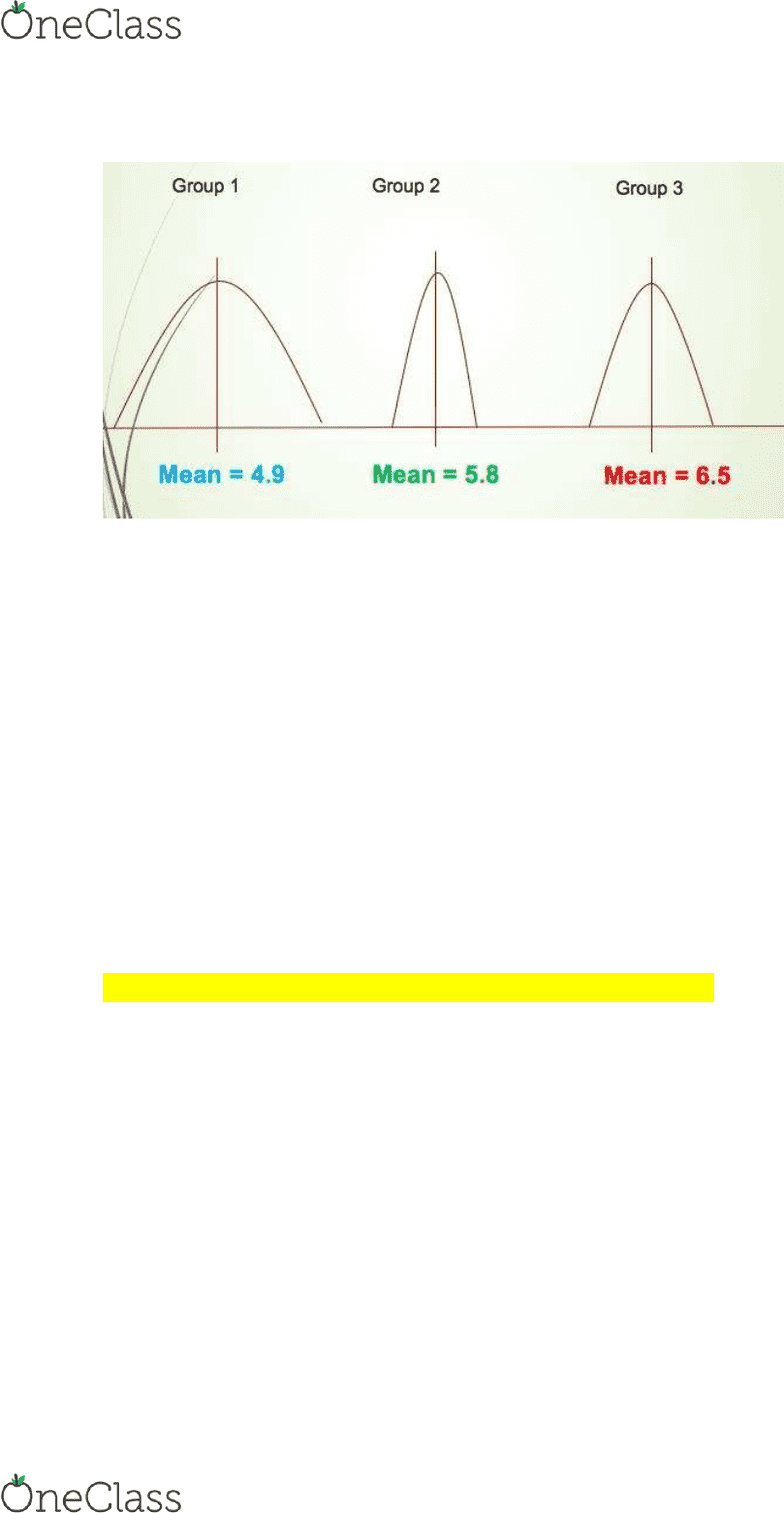# CRIM 320 Lecture Notes - Lecture 8: Null Hypothesis, Statistical Hypothesis Testing, Dependent And Independent Variables

86 views8 pages
School
Department
Course
ProfessorLecture 7: Analysis of Variance ANOVA
-ANOVA best thought of as an extension of t tests
-like t tests, ANOVA starts by comparing differences in means between groups
-also comparing sample means
-what happens when your grouping variable has more than two categories?
-comparing group means of an outcome variable of interest to determine these means are
significantly different from each other
-today, focus on one-way ANOVA
-comparing group means when we have more than 2 independent groups
-categories on a variable, 3 or more groups
the logic of ANOVA
-variance is spread of our data
-within-group variability
-spread of the data around the mean of each group
-how observations within each group vary
-between-group variability
-each group will have their own mean score on the outcome
-group means may be equal or difference
-how much our group means vary from each other
-based on these two estimates of variability, draw conclusions about the population means
-are they significantly different from each other?
what is the purpose of different categories?
-to group like things together
-similar scores on an outcome
within-group variability - spread around the mean
-group 1 is the most spread out around the mean (most difference)
-group 2 is small distribution, more clustered around the mean
find more resources at oneclass.com
find more resources at oneclass.com
Unlock document

This preview shows pages 1-3 of the document.
Unlock all 8 pages and 3 million more documents.between-group variability - each group will have a mean score
-each group will have own mean score
-how each mean values vary between groups
the procedure
-first question: are there differences in means across the groups?
-usually there is some difference in means
-are they statistically significant or not
-chance: are observed differences attributable to random variability in the sample?
-not statistically different
OR
-true difference: is there reason to believe that someone of the groups have different values in
the population?
-statistically different
-large enough we would expect them to be real relationships in the actual population
hypothesis testing
-null hypothesis: the population means for all groups is the same
-there are no differences between group mean scores on the dependent variable
-alternative hypothesis: there are differences between groups
-mean score on dependent variable
-note: the alternative hypothesis does not state which group differences are significant
-ANOVA on its own only tells if one of groups are significantly different from another group but
will not tell which group is different from each other
-use post hoc comparison tests
one-way ANOVA
-called one-way because it utilizes one grouping variable
-categorical variable with three of more categories (i.e., groups)
-interested in comparing in terms of mean score on their outcome
-grouping variable is often referred to a factor
find more resources at oneclass.com
find more resources at oneclass.com
Unlock document

This preview shows pages 1-3 of the document.
Unlock all 8 pages and 3 million more documents.-factors will normally be nominal level of measurement
-dependent variable will be interval or ratio level of measurement
assumptions
-1. Dependent variable is normally distributed for each of the populations as defined by the
different levels of the factor
-requires testing for normality
-dependent variable is not skewed or chaotic
-2. The variances of the dependent variable are the same for all populations
-requires usig Leee’s test
-3. The cases are obtained via random sampling
-4. The cases are independent of one another
how does ANOVA test for group differences?
-calculate the F ratio
-F = between-groups mean square / within-groups mean square
-requires calculating the between- and within-group variability
-sum of squares and degree of freedom for within-group and separately for between-group
estimating within-group variability I
-1. Compute within-groups sum of squares
-sum of our squared standard deviations from group means
-a) obtain variance for each group (SD2)
-b) multiple each variance by (group N-1)
-c) sum up
-2. Compute the degrees of freedom
-sum of (group N-1) for all groups
-have two different degrees of freedom
-3. Divide the sum of squares by its degrees of freedom
-4. WMS within mean square
-average of the variances in each of the groups, adjusted for the number of observations
in each group (how spread out our scores are)
*know bolded points for exam
estimating between-group variability I
-1. Compute between-groups sum of squares
-a) subtract overall mean from each group mean
-b) square each of these differences
-c) multiply the square by number in each group
-d) sum up
-2. Compute the degrees of freedom
-number of groups 1
-3. Divide the sum of squares by its degrees of freedom
find more resources at oneclass.com
find more resources at oneclass.com
Unlock document

This preview shows pages 1-3 of the document.
Unlock all 8 pages and 3 million more documents.

# Get access

\$10 USD/m
Billed \$120 USD annually
Homework Help
Class Notes
Textbook Notes